Courses
Courses for Kids
Free study material
Free LIVE classes
More

# NCERT Solutions for Class 10 Maths Chapter 10 - ExerciseLIVE
Join Vedantu’s FREE Mastercalss

## NCERT Solutions for Class 10 Maths Chapter 10 Circles (Ex 10.2) Exercise 10.2

NCERT Solutions Class 10 Chapter 10 Ex.-10.2 is on Circles. This chapter on circles has 4 major parts that need to be covered for a solid understanding of this topic. The 4 important topics that are covered in this chapter on Circles are tabulated below. We advise students to go through these topics carefully to gain a precise understanding of the overall chapter.

 Sl. No. Topics 1 An Introduction 2 Tangent to a Circle 3 Number of Tangents from a Point on a Circle 4 A Summary

### Importance of Circles in Mathematics

To put it in simple words, a circle is a round two-dimensional figure consisting of infinitely many points on its circumference that are all equal in distance from the centre of the circle.

Circles are important in mathematics. Circles form a huge part of the geometry syllabus and there are many theorems related to the study of circles and their properties. This chapter is an elaborate one and we highly recommend that students should go through all the topics covered in it and the NCERT solutions we have provided to get a good grasp on the topic. Thus, they will be able to solve problems that are based on circles. Practising these questions will ensure a greater possibility to score well in exams.

Last updated date: 01st Oct 2023
Total views: 513k
Views today: 14.13k
Watch videos on
NCERT Solutions for Class 10 Maths Chapter 10 - ExerciseCircles [ Full Chapter ] in One Shot | CBSE Class 10 Maths Chapter 10 | Umang 2021 | Term 2 Vedantu
Vedantu 9&10
SubscribeShare
17K likes
356.4K Views
1 year ago
Play QuizCircles L-2 (Number Of Tangents From A Point Outside the Circle) CBSE Class10 Maths | Term2 Vedantu
Vedantu 9&10
3.6K likes
72K Views
1 year agoCircles L-1 [ Introduction & Tangents to a Circle ] CBSE 10 Maths Chapter 10 [Term 2 Exam] Vedantu
Vedantu 9&10
6.5K likes
165K Views
1 year ago
Play QuizCircles in One Shot | CBSE Class 10 Maths Chapter 10 NCERT Solutions | Vedantu Class 9 and 10
Vedantu 9&10
8.8K likes
195.2K Views
2 years ago
Play Quiz
See More## Access NCERT Solutions for Class 10 Mathematics Chapter 10 – Circles

Exercise 10.1

1. How many tangents can a circle have?

Ans:

We know that a circle has an infinite number of points on its perimeter. Hence, there will be an infinite number of tangents on a circle.

2. Fill in the blanks:

i. A tangent to a circle intersects it in _________ point(s).

Ans:

A tangent to a circle intersects it in exactly one point(s).

ii. A line intersecting a circle in two points is called a _______.

Ans:

A line intersecting a circle in two points is called a secant.

iii. A circle can have _______ parallel tangents at the most.

Ans:

A circle can have two parallel tangents at the most.

iv. The common point of a tangent to a circle and the circle is called ______.

Ans:

The common point of a tangent to a circle and the circle is called point of contact.

3. A tangent $PQ$ at a point $P$ of a circle of radius $5\ \text{cm}$ meets a line through the centre $O$ at a point $Q$ so that $OQ=12\ \text{cm}$.

Length of $PQ$ is: (A) $12\ \text{cm}$ (B) $13\ \text{cm}$ (C) $8.5\ \text{cm}$ (D) $\sqrt{119}$.

Ans:

As, the tangent $PQ$ is base and radius is the height of $5\ \text{cm}$. Also, $OQ$ is the hypotenuse. Therefore, the length of $PQ$ will be –

$PQ=\sqrt{O{{Q}^{2}}-O{{P}^{2}}}$

$\Rightarrow PQ=\sqrt{{{12}^{2}}-{{5}^{2}}}$

$\Rightarrow PQ=\sqrt{144-25}$

$\Rightarrow PQ=\sqrt{119}$

Hence, option $(D)$ is correct.

4. Draw a circle and two lines parallel to a given line such that one is tangent and the other, a secant to the circle.

Ans:

From the figure given above let us assume that a line $l$ and a circle having centre $O$, comprises a line $PT$ which is parallel to the line $l$ and is a tangent to the circle. Similarly, $AB$ is a secant parallel to the line $l$.

Exercise 10.2

1. From a point $Q$, the length of the tangent to a circle is $24\ \text{cm}$ and the distance of $Q$ from the centre is $25\ \text{cm}$. The radius of the circle is

(A) $7\ \text{cm}$ (B) $12\ \text{cm}$ (C) $15\ \text{cm}$ (D) $24.5\ \text{cm}$.

Ans: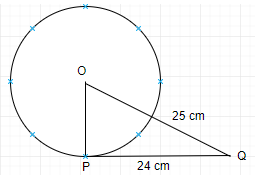From the figure given above, let us assume that $O$ is the centre of the circle and given we have $PQ$ as the tangent to the circle of length $24\ \text{cm}$, and $OQ$ is of length $25\ \text{cm}$.

Now, by using the Pythagoras theorem we have –

$OP=\sqrt{O{{Q}^{2}}-P{{Q}^{2}}}$

$\Rightarrow OP=\sqrt{{{25}^{2}}-{{24}^{2}}}$

$\Rightarrow OP=\sqrt{625-576}$

$\Rightarrow OP=\sqrt{49}$

$\Rightarrow OP=7\ \text{cm}$.

Hence, option $(A)$ is correct.

2. In the given figure, if $TP$ and $TQ$ are the two tangents to a circle with centre $O$ so that $\angle POQ=110{}^\circ$, then $\angle PTQ$ is equal to

(A) $60{}^\circ$ (B) $70{}^\circ$ (C) $80{}^\circ$ (D) $90{}^\circ$.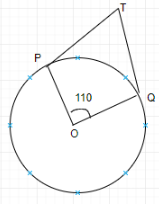Ans:

As, from the given figure, we can observe that there are two tangents perpendicular to the radius of the circle as $TP$ and $TQ$. Since, they are perpendicular to the radius hence, we have $\angle OPT=90{}^\circ$ and

$\angle OQT=90{}^\circ$.

Therefore, $\angle PTQ=360{}^\circ -\angle OPT-\angle OTQ-\angle POQ$

$\Rightarrow \angle PTQ=360{}^\circ -90{}^\circ -90{}^\circ -110{}^\circ$

$\Rightarrow \angle PTQ=360{}^\circ -290{}^\circ$

$\Rightarrow \angle PTQ=70{}^\circ$

Hence, option $(B)$ is correct.

3. If tangents $PA$ and $PB$ from a point $P$ to a circle with centre $O$ are inclined to each other an angle of $80{}^\circ$, then $\angle POA$ is equal to

(A) $50{}^\circ$ (B) $60{}^\circ$ (C) $70{}^\circ$ (D) $80{}^\circ$.

Ans: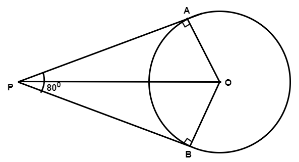As, we have two tangents $PA$ and $PB$ which will be perpendicular to the radius of the circle. Hence, we have $\angle PAO=90{}^\circ$ and

$\angle PBO=90{}^\circ$. Since this is a quadrilateral, we will have the sum of all interior angles equal to $360{}^\circ$.

Therefore,

$\angle PAO+\angle PBO+\angle APB+\angle AOB=360{}^\circ$

$\Rightarrow 90{}^\circ +90{}^\circ +80{}^\circ +\angle AOB=360{}^\circ$

$\Rightarrow \angle AOB=360{}^\circ -260{}^\circ$

$\Rightarrow \angle AOB=100{}^\circ$.

Now, we know that $\angle POA$ is half of the $\angle AOB$.

Therefore,

$\angle POA=\frac{\angle AOB}{2}$

$\Rightarrow \angle POA=\frac{100{}^\circ }{2}$

$\Rightarrow \angle POA=50{}^\circ$.

Hence, option $(A)$ is correct.

4. Prove that the tangents drawn at the ends of a diameter of a circle are parallel.

Ans:As, from the figure given above, let us assume that $O$ is the centre of the circle and $AB$, $CD$ are the two tangents at the ends of the diameter of the circle.

Now, we know that tangents are perpendicular to the radius of the circle.

Hence, $\angle CQO=90{}^\circ$

$\angle DQO=90{}^\circ$

$\angle APO=90{}^\circ$

$\angle BPO=90{}^\circ$.

Therefore, we can say that $\angle CPQ=\angle BQP$ because they are alternate angles. Similarly, $\angle AQP=\angle QPD$.

Hence, if the interior alternate angles are equal then the lines $AB$, $CD$ should be parallel lines.

Hence, proved.

5. Prove that the perpendicular at the point of contact to the tangent to a circle passes through the centre.

Ans: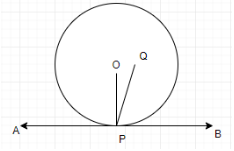Let us assume that the line perpendicular at the point of contact to the tangent of a circle does not pass through the centre $O$ but passes through a point $Q$ as shown in the figure above.

Hence, we have the line $PQ\bot AB$.

$\Rightarrow \angle QPB=90{}^\circ$

Also, we have $\angle OPB=90{}^\circ$.

After comparing both the equations we get –

$\Rightarrow \angle QPB=\angle OPB$

But, from the figure drawn above we can observe that this is not the case as $\angle QPB<\angle OPB$.

Therefore, we can conclude that $\angle QPB\ne \angle OPB$. They can only be equal when these two-line segments $QP$ and $OP$ will be equal. This implies that the line perpendicular at the point of contact to the tangent of a circle passes through the centre $O$. Hence, proved.

6. The length of a tangent from a point $A$ at distance $5\ \text{cm}$ from the centre of the circle is $4\ \text{cm}$. Find the radius of the circle.

Ans: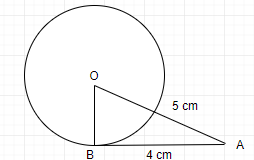From the figure given above, let us assume that $O$ is the centre of the circle and given we have $AB$ as the tangent to the circle of length $4\ \text{cm}$, and $OA$ is of length $5\ \text{cm}$.

Now, by using the Pythagoras theorem we have –

$OB=\sqrt{O{{A}^{2}}-A{{B}^{2}}}$

$\Rightarrow OB=\sqrt{{{5}^{2}}-{{4}^{2}}}$

$\Rightarrow OB=\sqrt{25-16}$

$\Rightarrow OB=\sqrt{9}$

$\Rightarrow OB=3\ \text{cm}$.

Therefore, the radius of the circle will be $3\ \text{cm}$.

7. Two concentric circles are of radii $5\ \text{cm}$ and $3\ \text{cm}$. Find the length of the chord of the larger circle which touches the smaller circle.

Ans: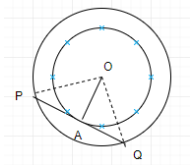From the figure given above, we can observe that the line segment $PQ$ is the chord of the larger circle and a tangent to the smaller circle.

Therefore, $OA\bot PQ$.

Now, we can observe that the $\Delta OAP$ forms a right-angled triangle.

Hence, by applying Pythagoras theorem –

$AP=\sqrt{O{{P}^{2}}-O{{A}^{2}}}$

$\Rightarrow AP=\sqrt{{{5}^{2}}-{{3}^{2}}}$

$\Rightarrow AP=\sqrt{25-9}$

$\Rightarrow AP=\sqrt{16}$

$\Rightarrow AP=4\ \text{cm}$.

Now, since the radius is perpendicular to the tangent therefore, we have $AP=AQ$.

Hence, $PQ=2AP$.

$\Rightarrow PQ=2\times 4$

$\Rightarrow PQ=8\ \text{cm}$.

So, the length of the chord will be of $8\ \text{cm}$.

8. A quadrilateral $ABCD$ is drawn to circumscribe a circle. Prove that $AB+CD=AD+BC$.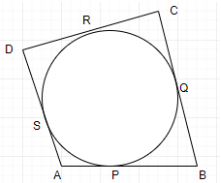Ans:

From the figure given we can observe that the sides of the quadrilateral acts as tangents to the circle. As, the tangents drawn from any external point will have the same length. Therefore, we have –

$DR=DS$,

$CR=CQ$,

$BP=BQ$, and

$AP=AS$.

Now, we will add all the relations.

Hence, $DR+CR+BP+AP=DS+CQ+BQ+AS$

$\Rightarrow \left( DR+CR \right)+\left( BP+AP \right)=\left( DS+AS \right)+\left( CQ+BQ \right)$

$\Rightarrow DC+AB=AD+BC$

Hence, proved.

9. In the given figure, $XY$ and $X'Y'$ are two parallel tangents to a circle with centre $O$ and another tangent $AB$ with point of contact $C$ intersecting $XY$ at $A$ and $X'Y'$ at $B$. Prove that $\angle AOB=90{}^\circ$.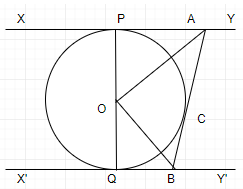Ans: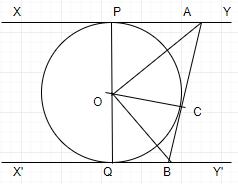From the figure given we can observe that $AB$, $XY$ and $X'Y'$ are tangents to the circle and will be perpendicular to its radius.

Now, let us consider two triangles $\Delta OPA$ and $\Delta OCA$, such that –

$OP=OC$,

$AP=AC$.

Hence, we have $\Delta OPA\cong \Delta OCA$ by the SSS congruence rule.

$\Rightarrow \angle POA=\angle COA$.

In the similar manner $\Delta OQB\cong \Delta OCB$.

$\Rightarrow \angle QOB=\angle COB$.

Now, we know that $PQ$ is the diameter of the circle.

$\Rightarrow \angle POA+\angle COA+\angle COB+\angle QOB=180{}^\circ$

$\Rightarrow 2\angle COA+2\angle COB=180{}^\circ$

$\Rightarrow \angle COA+\angle COB=90{}^\circ$

$\Rightarrow \angle AOB=90{}^\circ$.

Hence, proved.

10. Prove that the angle between the two tangents drawn from an external point to a circle is supplementary to the angle subtended by the line-segment joining the points of contact at the centre.

Ans: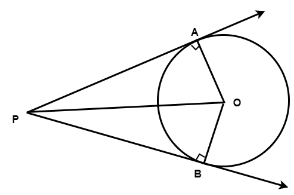Let us assume a circle with centre at $O$, which have two tangents $PA$ and $PB$ which are perpendicular to the radius of the circle as shown in the figure above.

Now, let us consider two triangles $\Delta OAP$ and $\Delta OBP$, such that –

$PA=PB$, and

$OA=OB$.

Hence, we have $\Delta OAP\cong \Delta OBP$ by the SSS congruence criteria.

Therefore, $\angle OPA=\angle OPB$ and

$\angle AOP=\angle BOP$.

This implies that $\angle APB=2\angle OPA$ and

$\angle AOB=2\angle AOP$.

Hence, in the right-angled triangle $\Delta OAP$, we have –

$\angle AOP+\angle OPA=90{}^\circ$

$2\angle AOP+2\angle OPA=180{}^\circ$

$\Rightarrow \angle AOB=180{}^\circ -\angle APB$

$\Rightarrow \angle AOP+\angle OPA=180{}^\circ$.

Hence, proved.

11. Prove that the parallelogram circumscribing a circle is a rhombus.

Ans: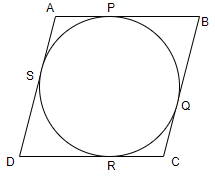From the figure given above, we can observe that $ABCD$ is a parallelogram whose sides are tangent to the circle. This implies that $DR=DS$,

$CR=CQ$,

$BP=BQ$, and

$AP=AS$.

Now, we will add all the relations.

Hence, $DR+CR+BP+AP=DS+CQ+BQ+AS$

$\Rightarrow \left( DR+CR \right)+\left( BP+AP \right)=\left( DS+AS \right)+\left( CQ+BQ \right)$

$\Rightarrow DC+AB=AD+BC$

As, the sides of a parallelogram are always parallel and equal in length.

Therefore, $AB=DC$ and

$AD=BC$.

$\Rightarrow 2AB=2BC$

$\Rightarrow AB=BC$

Hence, all the sides of parallelogram are equal.

Therefore, we can conclude that it is a rhombus. Hence, proved.

12. A triangle $ABC$ is drawn to circumscribe a circle of radius $4\ \text{cm}$ such that the Segments $BD$ and $DC$ into which $BC$ is divided by the point of contact $D$ are of lengths $8\ \text{cm}$ and $6\ \text{cm}$ respectively. Find the sides $AB$ and $AC$.

Ans: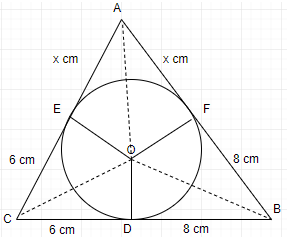From the figure given above, we can observe that the sides of a triangle $ABC$ are the tangents to the circle and are perpendicular to the radius of the circle.

Hence, in $\Delta ABC$

$CF=CD=6\ \text{cm}$

Similarly, $BE=BD=8\ \text{cm}$ and

$AE=AF=x\ \text{cm}$.

In $\Delta ABE$,

$AB=AE+BE$

$\Rightarrow AB=x+8$

Similarly, $BC=8+6=14$ and

$CA=6+x$.

Therefore, we have $2s=AB+BC+CA$

$\Rightarrow 2s=x+8+14+6+x$

$\Rightarrow 2s=2x+28$

$\Rightarrow s=x+14$.

Now, we know that the area of a triangle can be calculated by using the formula $Area=\sqrt{s(s-a)(s-b)(s-c)}$.

Therefore,

$Area\ \text{of}\ \Delta \text{ABC}=\sqrt{(14+x)(14+x-6-x)(14+x-8-x)}$

$\Rightarrow \sqrt{x(14+x)(8)(6)}$

$\Rightarrow 4\sqrt{3(14x+{{x}^{2}})}$.

Hence, $Area\ \text{of}\ \Delta \text{OBC = }\frac{1}{2}\times OD\times BC$

$Area\ \text{of}\ \Delta \text{OBC =}28$

$\Rightarrow Area\ \text{of}\ \Delta \text{OCA = }\frac{1}{2}\times OF\times AC$

$Area\ \text{of}\ \Delta \text{OCA = 12+2x}$

$\Rightarrow Area\ \text{of}\ \Delta \text{OAB = }\frac{1}{2}\times OE\times AB$

$Area\ \text{of}\ \Delta \text{OAB = 16+2x}$

Therefore, the total area of the triangle $ABC$ will be –

$=Area\ \Delta \text{OBC}+Area\ \Delta \text{OCA+}Area\ \Delta \text{OAB}$

$\Rightarrow 4\sqrt{3(14x+{{x}^{2}})}=28+12+2x+16x+2x$

$\Rightarrow \sqrt{3(14x+{{x}^{2}})}=14+x$

$\Rightarrow 3(14x+{{x}^{2}})={{(14+x)}^{2}}$

Hence, after further solving we have –

$(x+14)(x-7)=0$

$\Rightarrow x=-14$ or

$\Rightarrow x=7$

As, the side cannot be negative in nature, therefore, $x=7\ \text{cm}$.

Hence, $AB=7+8$

$\Rightarrow AB=15\ \text{cm}$ and

$CA=6+7$

$\Rightarrow CA=13\ \text{cm}$.

13. Prove that opposite sides of a quadrilateral circumscribing a circle subtend Supplementary angles at the centre of the circle.

Ans: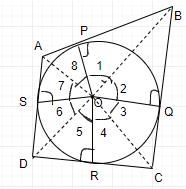From the figure given we can observe that the sides of the quadrilateral acts as tangents to the circle. Therefore, we have to prove that–

$\angle AOB+\angle COD=180{}^\circ$ and

$\angle BOC+\angle DOA=180{}^\circ$

Now, let us consider two triangles $\Delta OAP$ and $\Delta OAS$,

Hence, we have $AP=AS$, and

$OP=OS$

$\Rightarrow \Delta OAP\cong \Delta OAS$ by the SSS congruence criteria.

Thus, $\angle POA=\angle AOS$

$\angle 7=\angle 8$

Also,

$\angle 1=\angle 2$,

$\angle 3=\angle 4$,

$\angle 5=\angle 6$

Therefore, $\angle 1+\angle 2+\angle 3+\angle 4+\angle 5+\angle 6+\angle 7+\angle 8=360{}^\circ$

$\Rightarrow \left( \angle 1+\angle 2 \right)+\left( \angle 4+\angle 3 \right)+\left( \angle 6+\angle 5 \right)+\left( \angle 8+\angle 7 \right)=360{}^\circ$

$\Rightarrow 2\angle 1+2\angle 4+2\angle 5+2\angle 8=360{}^\circ$

$\Rightarrow \left( \angle 1+\angle 8 \right)+\left( \angle 4+\angle 5 \right)=180{}^\circ$

$\Rightarrow \angle AOB+\angle COD=180{}^\circ$

In the similar manner we can prove that $\angle BOC+\angle DOA=180{}^\circ$.

Therefore, we have proved that opposite sides of a quadrilateral circumscribing a circle subtend supplementary angles at the centre of the circle.

### NCERT Solutions for Class 10 Maths Chapter 10 Circles Exercise 10.2

Opting for the NCERT solutions for Ex 10.2 Class 10 Maths is considered as the best option for the CBSE students when it comes to exam preparation. This chapter consists of many exercises. Out of which we have provided the Exercise 10.2 Class 10 Maths NCERT solutions on this page in PDF format. You can download this solution as per your convenience or you can study it directly from our website/ app online.

Vedantu in-house subject matter experts have solved the problems/ questions from the exercise with the utmost care and by following all the guidelines by CBSE. Class 10 students who are thorough with all the concepts from the Subject Maths textbook and quite well-versed with all the problems from the exercises given in it, then any student can easily score the highest possible marks in the final exam. With the help of this Class 10 Maths Chapter 10 Exercise 10.2 solutions, students can easily understand the pattern of questions that can be asked in the exam from this chapter and also learn the marks weightage of the chapter. So that they can prepare themselves accordingly for the final exam.

Besides these NCERT solutions for Class 10 Maths Chapter 10 Exercise 10.2, there are plenty of exercises in this chapter which contain innumerable questions as well. All these questions are solved/answered by our in-house subject experts as mentioned earlier. Hence all of these are bound to be of superior quality and anyone can refer to these during the time of exam preparation. In order to score the best possible marks in the class, it is really important to understand all the concepts of the textbooks and solve the problems from the exercises given next to it.

Do not delay any more. Download the NCERT solutions for Class 10 Maths Chapter 10 Exercise 10.2 from Vedantu website now for better exam preparation. If you have the Vedantu app in your phone, you can download the same through the app as well. The best part of these solutions is these can be accessed both online and offline as well.

### NCERT Solutions for Class 10 Mathematics Chapter 10 Exercises

 Chapter 10 - Circles Exercises in PDF Format Exercise 10.1 4 Questions & Solutions (2 Short Answer, 2 Long Answers)

## FAQs on NCERT Solutions for Class 10 Maths Chapter 10 - Exercise

1. How many examples and questions are there in Exercise 10.2 of Class 10 Maths?

There are 5 examples involving 10.2 and its subsequent subtopics. Apart from that at the end of section 10.2, you would find Exercise 1 which will consist of a total of 14 questions arranged well in a hierarchy of difficulty. Practice all of these to get a good idea of the concepts mentioned in section 10.2.

2. Is Exercise 10.2 of Class 10 Maths easy?

Maths is enjoyable if you understand the methods, but if you are unfamiliar with the themes and formulas, things may get difficult and perplexing because there are various ways to answer a question or problem. Mathematics is a big topic, and it's easy to become lost in all of the formulae and derivations. Concentrate on the basics, and with enough practise, you'll be fine.

3. Where can I find the solution to Class 10 Maths Chapter 10?

To get the solutions of NCERT Class 10 Maths Chapter 10, you can go and visit the Vedantu site and search for the solutions of Class 10 Maths Chapter 10. Apart from this you can avail multiple modules and help to crack good scores in math exams. The link for the solutions to the exercise is NCERT Class 10 Maths Chapter 10. Click on that to get a free of cost PDF version of the solutions.

4. Is it compulsory to solve all examples and exercises of NCERT Class 10 Maths Chapter 10?

You definitely must practice each one of them as you never know what concept you might be facing difficulty in unless you test your knowledge by solving different types of questions and problems. Hence it is of utmost importance that you solve all examples and exercises of NCERT Class 10 Maths Chapter 10. Revise all the concepts and practice as many problems as you can to grasp the concepts better. Also, visit the Vedantu site for more problems regarding these topics.

5. How many Chapters are there in the NCERT Solutions Class 10 Maths apart from Chapter 10?

The NCERT Class 10 Maths textbook has 16 chapters as well as some selected problems for each topic. Each chapter must be given equal weight in order to have a comprehensive grasp of all of the topics provided for Class 10 students and to perform well in your examinations. If you are interested in obtaining various modules and example papers relating to these areas, you can simply get them through the Vedantu website as well as the Vedantu mobile app.